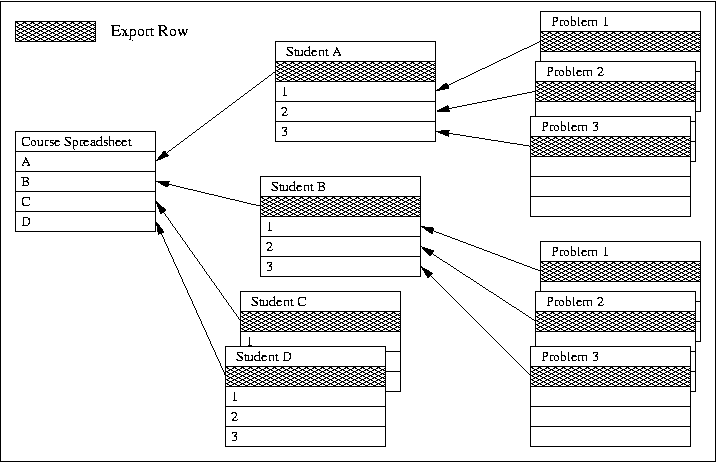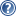###LON-CAPA Help

Each Student and Assessment level spreadsheets permit sophisticated calculations. The summary values that are to be passed to the parent spreadsheet are summarized in the export row of the sheet. The figure shows the spreadsheet hierarchy and indicates the flow of information.1. The Course Spreadsheet

The course spreadsheet contains all of the summary data for all the students in the course.

2. The Student Spreadsheet

The student level spreadsheet summarizes the assessment spreadsheet data for the students. There is only one default student level spreadsheet definition, but the data varies for each student. The student spreadsheet is programmed to permit the instructor to assign different assessment spreadsheets for different grade categories or other uses. The data present in the student spreadsheet is imported from the export row () of each assessment spreadsheet for all of the assessments currently in the course. The student spreadsheets pass summary data to the course-level spreadsheet via the student spreadsheet export row ().

3. The Assessment Spreadsheets

An assessment-level spreadsheet provides access to all of the parameters associated with a student's performance on a particular homework assignment, quiz, or exam. Each student has an assessment spreadsheet for every assessment in the course. The assessment spreadsheets provide data to their parent, the student level spreadsheet, via the assessment spreadsheet export row (). Multiple assessment spreadsheets can be used in a course, facilitating organizing assessments into categories or for other purposes.